# 第六章 积 分 学

## §１单变量函数的积分

### 一、积分基本概念

［不定积分原函数 如果在给定的区间[a ,b]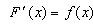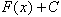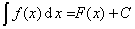`表示f(x)的原函数全体，称为f(x)的不定积分.`

［定积分·黎曼积分］ 设在区间[a,b]上给定了函数f(x).用任意方法把区间[a,b]分成若干部分，其分点为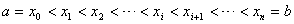，并设λ是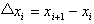(i=0,1,2,,n1)中最大的.在每一个小区间[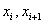]上任取一点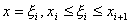(i=0,1,2,,n1),作和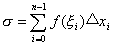6.1.λ0时，如果极限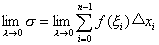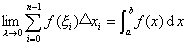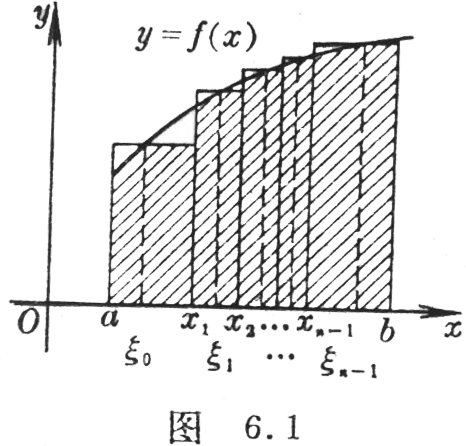［牛顿－莱布尼茨公式］ 若函数f(x)在区间[a,b]上连续，或分段连续，则f(x)[a,b]上有原函数，设F(x)f(x)[a,b]上的一个原函数，则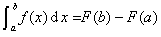［可积函数及其性质］

(１) 若函数f(x)在区间[a,b]上连续，则f(x)是可积的.

(２) 若函数f(x)[a,b]上有界，且只有有限多个间断点，则f(x)是可积的.

(３) 单调有界函数一定是可积的.

(４) 可积函数一定是有界的.

(５) 若函数f(x)可积，则|f(x)|kf(x)k为常数）也可积.

(６) 若函数f(x)g(x)可积，则其和、差、乘积也可积.

(７) 若函数f(x)[a,b]上可积，则f(x)[a,b]中的任一部分区间[α,β]上也可积.反之，若把[a,b]分割成若干部分区间，并分别在每个部分区间上f(x)可积，则它在整个区间[a,b]上可积.

［积分中值定理］儍儍儍儍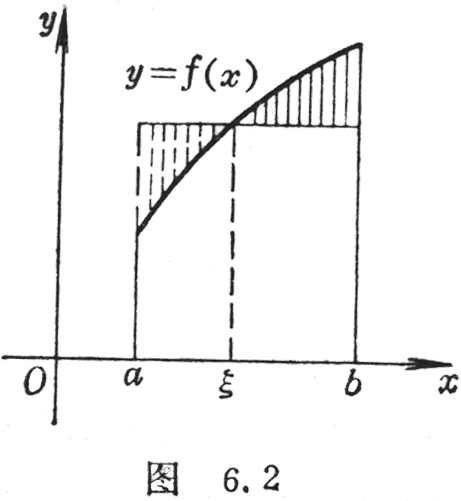(１) 若函数f(x)在区间[a,b]上连续（图6.2），则在区间[a,b]内至少存在一个数ξ(a<ξ<b)，使得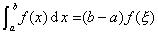(２) 若函数f(x)g(x)在区间[a,b]上有界且可积，f(x)连续，g(x)在区间[a,b]内不变号，则在区间[a,b]内至少存在一个数ξ(a<ξ<b)，使得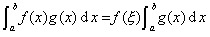(３) 若函数f(x)g(x)在区间[a,b]上有界且可积，而f(x)[a,b]上是单调的，则在区间[a,b]内至少存在一个数ξ(a<ξ<b)，使得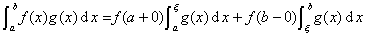(４) 除此条件而外，若f(x)非负单调下降广义的，则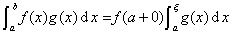(a<ξ<b)

f(x)非负单调上升广义的，则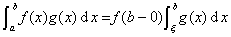(a<ξ<b)